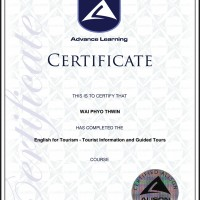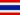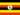# Lección 4 Introducción a programas de hoja de cálculo

• Apuntes
• Revisión por temaWAI P. 0 0 Lesson 4 - Introduction to Spreadsheet Programs Lesson Introduction and Objectives Introduction to Spreadsheet Programs Lesson Introduction Suppose you have to calculate the expenses of an entire month. You note the expenses for each day and calculate the total expenses for the month. You use a calculator to do the calculation, or you can manually add the figures. After you finish the calculation, you realize that some of the data that you used in the calculation was wrong. Now, all your time and effort is wasted, and you have to do the entire calculation again. If you use a spreadsheet, you can avoid these types of calculation problems, and save time and effort. A spreadsheet is a table in which you can store and organize data, and also perform numeric calculations. This makes your work easier because there is no need to perform calculations manually or with a calculator. It is easy to modify the data in a spreadsheet. When you modify the data used in a calculation, the result of the calculation is modified automatically. Therefore, you do not have to spend time or effort in recalculation. In this lesson, you will learn about the components of a spreadsheet and how to store data in it. You will also learn to perform basic mathematical tasks, and create charts and graphs in a spreadsheet. In addition, you will learn about the various options available for printing a spreadsheet. Lesson Objectives After completing this lesson, you will be able to: Identify the components of a spreadsheet. Enter data into a spreadsheet. Perform basic mathematical tasks in a spreadsheet. Insert charts in a spreadsheet. Print a spreadsheet.WAI P. 0 0 What is mean spreadsheet programs?Nsubuga Y. 0 0 COMPUTER KNOWLEDGE IS A CONCERN IN THE DAILY WORLD TECHNOLOGY GROWTH AND TO FIT IN THE MARKET OF TECHNOLOGY USES.Janeya Z. 0 0 it's useful.